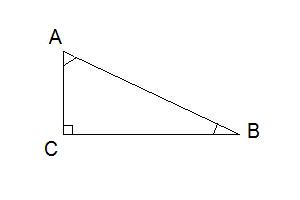# What is Trigonometry?

Try asking any number of young math students the question "what is trigonometry?"  Inevitably, you will learn that it is one of the most feared subjects in math that students have to learn.  However, solving these questions and equations is much easier than they often give it credit for.  (For some interesting background history and applications of this discipline in mathematics, wikipedia has a good article.)  On this page I'm going to go over some of the basics to get you started.  Some of my following posts will go into more details on the specific functions, so be sure to check those out as well.  And please remember to hit the Like button and/or +1 button if this helps you at all.

## Trigonometry Basics

Trigonometry, which literally means "triangle measurement," deals with the relationships between the angles and sides of triangles.  For the purposes of explaining the trigonometry basics, this is going to specifically deal with triangles that have one 90 degree angle (right-angle triangles).  These particular kinds of triangles have important distinctions to point out regarding their nomenclature.  The longest side, which is always the side that is directly opposite to the right angle, is called the HYPOTENUSE.  There is a common triangle notation of naming the sides and angles, as explained below, for which I will refer to this diagram:The first concept that is essential for you to really understand is how to name a triangle.  The standard convention of naming triangles is to name them by the letters of their 3 corners. So in this example, this is triangle ABC. Similarly, the sides are named by the corners at either end of the side, so here we have sides AB, BC, and AC. The angles may be named with a single letter, or designated by 3 letters representing the points that make up the angle, in order, with the corner point in the middle (ie. angle B = angle ABC, angle A = angle CAB, angle C = angle ACB).

An alternate way to look at triangles is to name the ANGLES with capital letters. Then, the side that is directly opposite the angle is given the same letter, but in lower case. Therefore, from the above example, side AC = side b, AB = c, and BC = a. Somewhat confusing, but not really... it makes sense. The keyword in this method of notation is OPPOSITE, which tells you that the angle and the side opposite to it are related.  If this doesn't quite make sense to you automatically, you can also consider that the side c is the only one that doesn't help make angle C.  Check it out and see for yourself that this concept applies to all of the angles in any triangle.  If you can understand this basic naming scheme used in triangle problems, solving trigonometry questions will be that much easier.

In working with triangle sides and angles, you will also need to be able to recognize their RELATIVE positions.  So, in addition to the hypotenuse, which can easily be identified, the other two sides can be labeled relative to the angles.  So, this means that for angle B, you could be interested to label its OPPOSITE side and ADJACENT side. The opposite side is easy to identify: it is the side that does not touch the corner you are looking at. The adjacent side equally easy, though care must be taken to not mistake it for the hypotenuse: it touches the corner, but is not the hypotenuse.  Also recognize that in a right angle triangle, the two acute angles will always be created by an adjacent side and the hypotenuse.

A solid understanding of these trigonometry basics, including triangle notations and the naming of angles and sides (in particular, the relative naming designations) is important before you can move on to confidently do calculations involving these measurements. These calculations in themselves, however, are very straightforward, and as will be explained in separate posts, may be figured out very easily by remembering just one word - SOHCAHTOA.  This is one of the most important key points there is to memorize on this topic!  Make sure you check out some of my other posts (specifically, my lessons about sine, cosine, and tangent, where you will find sohcahtoa explained simply).

I really hope that I'm doing a decent job at explaining trigonometry easily.  Please hit the Facebook Like or +1 buttons to recommend my post if you found any value in it, and leave me some feedback in the comments below - especially if I didn't answer "what is trigonometry" well enough for you.

#### 3 comments:

1.that is actually so helpful!
thankssss =]

2.really helpful

3.really helpfull but could do with gong in to the calculations of sohcahtoa! also maby a rhym like;
Silly Old Harry
Caught A Herring
Trawling Off America
to help people remember the word! apart form that its really GOOD!!
thank you it helped!!:)<3